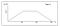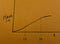# How Up-to-Date is our Analytics Warehouse?

## An over-the-top approach

In contrast with many analytics groups in the industry, Ro’s data analytics warehouse is updated close to something resembling “real time.” Ro’s Data team supports almost all departments in the company, including all operations team. As a simple example of real-time data for operations: the pharmacy operations team watches Looker dashboards all day to see if pharmacy order backlogs warrant an intervention; we promise 2-day delivery and we are good on our word!

The data team is often asked some variant of the question “How current is the data that I’m looking at?”

The answer is dependent on how many processes are required to get the data from its source to its destination. It may be anywhere from one process (a periodic syncing process) to several (simple example: periodic syncing process plus a periodic chain of processing tasks to refresh several linked derived tables). Abstractly, a zoom-out here will look like several processes whose timing is uncoupled and with each running periodically (ex: every ~15 minutes or so) but with no guarantee of starting or ending at a specific point on the hour. Essentially, data traveling to an access point in the data warehouse goes through a number of independently-timed processes, with each process recurring at a fixed rate.

Let’s take the example from above where two independent processes — A and B — run exactly every 10 and 20 minutes respectively; we’ll call the time intervals “period” (so periodA=10, periodB=20 respectively).

Let’s assume that processes A and B are nearly instantaneous once they begin; we can represent the time for new data to begin each process — and therefore complete it — as random variables A and B. Because each process really does occur only at exactly the specified intervals , the probability distribution of the wait time is the same as the probability of throwing a dart at a line that is period units long. In other words, the probability density function for the wait times is uniform from [0, period), with the y value being 1/period in that interval and 0 otherwise.

A and B are independent. The combined pdf of A+B can be found by convolving the two uniform distributions:Convolution of two uniform distributions, one from 0–10, the other from 0–20. Illustration found on probabilityexam.wordpress.com.

For a refresher on why convolving is the way to combine independent probability distributions, see this well-written wikipedia article.

Now that we have the probability density function of A+B, what we actually want is the cumulative density function of them, Pt(A+B). This answers the question “What is the probability that data makes it to the data warehouse within t minutes?” The graph of this cdf function will look vaguely like this:Pardon the graphics. Section 1 equation is y=x²/400; section 2 equation is y=(x-10)/20 + 1/4; section 3 is left up to the enterprising reader

In the above example, the most desirable/digestible answer to “How current is the data?” is actually usually to say something like “the data won’t be more than 30 mins old”. Simpler is better!

## Ro Data Team Blog

#### Ro Data Team Blog: data analytics, data engineering, data science